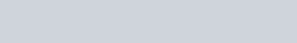Write the projection of the vector
Question:

Write the projection of the vector $(\hat{\mathrm{i}}+\hat{\mathrm{j}}+\hat{\mathrm{k}})$ along the vector $\hat{\mathrm{j}}$

Solution: